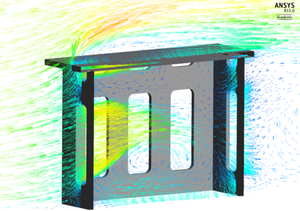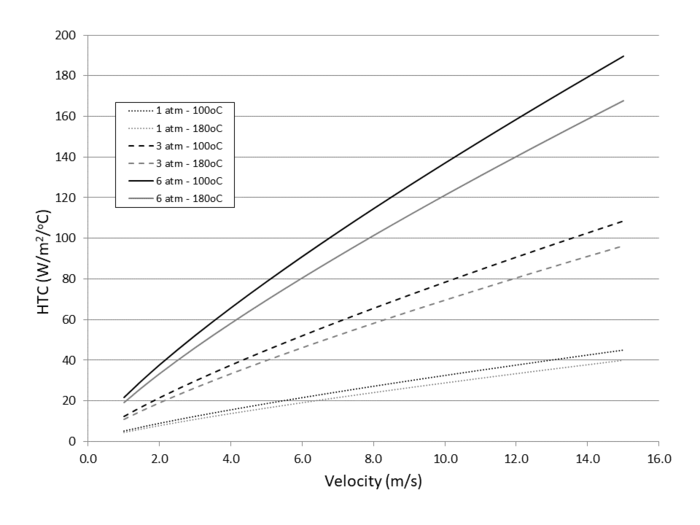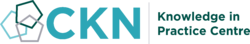The CKN Knowledge in Practice Centre is in the early stages of content creation and currently focuses on the theme of thermal management.
We appreciate any feedback or content suggestions/requests using the links below

# Convection - A106

Convection
Foundational knowledge articleDocument Type Article
Document Identifier 106
Themes
Relevant Class

Material

Tags
Prerequisites

## Introduction

Convective heat transfer, known as convection, is the heat transport mechanism associated with the flow of fluids acting as the carrier of thermal energy. It involves a combination of the sub-mechanisms of conduction and advection (bulk fluid motion). A classic physics example of convection is the heat transfer that occurs between a solid surface of a plate and fluid flowing over its surface. Locally, heat transfer occurs by conduction at the interface between the surface and the fluid, and away from the surface within the moving fluid . Together, this combined process forms the convective heat transfer process .

## Scope

This page explains the basics of thermal convection and puts the heat transfer mechanism in the context of composites processing.The difference between forced convection and natural convection is explained and the convective heat transfer coefficient (HTC), used to quantify this heat transfer mecahnism, is defined. Typical HTC values encountered in composite processes with factors that influence it are also given.

## Significance

Convection is the heat transfer mechanism between fluid (or gas) in contact with a solid surface. In composites processing, convection is an important heat transfer mechanism occurring in oven and autoclave processing methods. In these processes, heat is transferred between the hot gas environment to the composite part and tooling. This is also true for room temperature processing methods where convective heat transfer is taking place between the curing part/tool and the ambient air (gas) environment of the room.

## Prerequisites

Recommended documents to review before, or in parallel with this document:

## Convection Overview

Heat transfer by convection between a fluid and a solid surface is expressed by Newton’s law of cooling  (the same expression applies for heating):

$$q = h\, \Delta T$$ Newton’s law of cooling, where:<br />$$q$$ = Heat flux [W/m<sup>2</sup>],<br />$$h$$ = Convective heat transfer coefficient (HTC) [W/m<sup>2</sup>·K], and<br />$$\Delta T$$ = Temperature difference between fluid and solid surface [K],<br />

The above expression is sometimes expressed as a heat rate – where surface area is explicitly part of the expression:

$$\dot{Q} = h\, A\, \Delta T$$ Heat rate due to convection, where:<br />$$\dot{Q}$$ = Heat rate [W],<br />$$h$$ = Convective heat transfer coefficient (HTC) [W/m<sup>2</sup>·K],<br />$$A$$ = Surface area of solid surface [m<sup>2</sup>], and<br />$$\Delta T$$ = Temperature difference between fluid and solid surface [K],<br />

A key parameter in the above expressions is the convective heat transfer coefficient (HTC). The HTC depends on the fluid-flow conditions, thermophysical properties of the fluid, and flow path .

The HTC value quantifies the rate of heat transfer from the solid surface to the fluid (through the fluid film boundary layer). The greater the HTC value, the higher the heat transfer between the fluid and solid body surface.

## Mechanisms

A further differentiation of convective heat transfer is made between two of the possible fluid flow conditions:

1. Forced convection – where fluid flow is caused by some external means (e.g. a blowing fan), and
2. Natural convection – where fluid motion occurs naturally from the localized spatial temperature gradient inducing localized density changes within the fluid and a subsequent fluid motion.

### Forced convection

Forced convection implies a mechanically induced fluid flow and is the scenario encountered fluid motion is caused by external means such as fan, blower, pump or wind , causing an external pressure difference in the fluid . As shown in the earlier table, forced convection results in a much higher convective heat transfer coefficient (HTC) value and improved heat transfer. Simply put, the forced fluid flow improves the heat transfer process between the fluid and the solid surface.

Examples of forced convection situations in composites processing are fan blown ovens (convection ovens) and autoclave heating equipment. The blown gas over the curing part is beneficial, improving the heating and cooling processes.

### Natural convection

In natural or free convection, the fluid motion is purely from gravity and the natural fluid circulation due to the hot and cold fluid mixing. Fluid close to the heat source reduces in density and rises, and is subsequently replaced with surrounding colder and denser fluid. The resulting convective heat transfer coefficient (HTC) in natural convection scenarios is much lower than the HTC in forced convection. As a result, the heat transfer ability between the fluid and solid surface is reduced when compared to the mechanically induced forced convection scenario.

In composites processing, natural convection is encountered with an oven without a blown fan (natural convection oven), and room temperature curing where the gas motion of the ambient environment is occurring naturally.

### Effect on heat transfer coefficient

The effects of fluid flow on convective heat transfer is shown in the example HTC ranges provided below for different gasflow conditions, representing natural convection and forced convection conditions (source: ).

Gasflow Condition HTC

[W/m2·K]

HTC

[BTU/ft2·hr·°F]

Stagnant Gas, Natural Convection 5-25 1-4
Forced Convection, air/gas (1 atm) 10-250 2-44

While the above table of values show that a HTC as high as 250 W/m2·K can be obtained with forced convection gasflow (at 1 atm pressure), the expected HTC in composites processing are typically lower. This is discussed in the next section.

## Convective Heat Transfer in Composites Processing

Convective heat transfer is dependent on any factor influencing the properties of the heat transfer fluid and its flow path. In composites processes using oven or autoclave curing where hot gas (air or nitrogen) is the fluid, these factors include:

• Gas velocity
• Gas pressure
• Gas temperature
• Laminar or turbulent flow
• Surface orientation
• Surface geometry

### Effect of: gas velocity, pressure, and temperature

The first of the 3 factors on the above list (gas velocity, gas pressure, gas temperature) are regulated by the heating equipment. Their influence on convective heat transfer will be briefly discussed here.

With the capability limits of the heating equipment, and the material manufacturer defined curing conditions for the matrix; the influencing factors of imposed gas velocity, pressure and temperature fall within a limited range for composites processing. As a result, HTC values under 200 W/m2·K are typically observed for autoclave processing, and a lower HTC is observed for oven processing. In the following figure, the effects of gas velocity, pressure, and temperature on the convective heat transfer coefficient (HTC) within the bounds that can be achieved in autoclave processing are shown.Correlation of the convective heat transfer coefficient ($$h$$) as a function of the average gas velocity for a fully turbulent flow over a flat plate (calculated for a characteristic flow length of 1 m). Source:  .

The curves at 1 atm pressure can be considered the behaviour obtained in a forced convection oven. While the applied pressure results of 3 atm and 6 atm represent the applied pressure that can provided by an autoclave. Depending on the applied process conditions in the autoclave, the convective heat transfer HTC varies, where it can range from as low as 10 W/m2·K to nearly as high as approaching 200 W/m2·K. At applied pressure conditions, a significant sensitivity to gas velocity is observed, where an increase in the gas velocity has a corresponding large influence on dramatically increasing the effective HTC.

As illustrated in the above example, the following effects can be summarized:

Factor HTC Outcome Notes:
Increasing Gas Velocity Increasing Convective HTC
• Influence increases dramatically with at higher gas pressures
Increasing Gas Pressure Increasing Convective HTC
• Applied pressure is limited to 6-7 atm in most conventional autoclaves
Increasing Temperature Decreasing Convective HTC
• Curing temperature of typical thermosets (e.g. epoxy) is around 200°C or lower

As summarized in the above table, some factors that can be imposed like pressure or gas velocity can improve the convective heat transfer, while other necessary factors like increasing temperature that required for matrix curing, decreases the convective heat transfer effect. For autoclave processing, a simple relationship was observed for turbulent gas flow by Johnston et al. .

$$h \varpropto \left ( \frac{P}{T} \right )^{\frac{4}{5}}$$ Relationship between pressure, temperature and convective heat transfer coefficient for turbulent flow:<br />$$h$$ = Convective heat transfer coefficient (HTC),<br />$$P$$ = Pressure, and<br />$$T$$ = Temperature

### Effect of: surface orientation and surface geometry

The surface orientation and geometry of the tool-part assembly may play a significant role in blocking or redirecting gas flow in a convective heating environment. In turn, this may alter the boundary layer across the tool/part, thereby altering the laminar and turbulent flow regions, and thus the HTC. The impact the tool and part(s) have on flow are heavily dependent on the their placement within the heating environment. To learn more about how the tool/part may influence gas flow, click on the links above.The tool-part assembly may block or redirect gas flow in a forced convection environment.

## Related pages

Introduction to Composites Articles
Foundational Knowledge Articles
Foundational Knowledge Method Documents
Foundational Knowledge Worked Examples
Systems Knowledge Articles
Systems Knowledge Method Documents
Systems Knowledge Worked Examples
Systems Catalogue Articles
Systems Catalogue Objects – Material
Systems Catalogue Objects – Shape
Systems Catalogue Objects – Tooling and consumables
Systems Catalogue Objects – Equipment
Practice Documents
Case Studies
Perspectives ArticlesAbout Help## Welcome

Welcome to the CKN Knowledge in Practice Centre (KPC). The KPC is a resource for learning and applying scientific knowledge to the practice of composites manufacturing. As you navigate around the KPC, refer back to the information on this right-hand pane as a resource for understanding the intricacies of composites processing and why the KPC is laid out in the way that it is. The following video explains the KPC approach:

## Understanding Composites Processing

The Knowledge in Practice Centre (KPC) is centered around a structured method of thinking about composite material manufacturing. From the top down, the heirarchy consists of:

The way that the material, shape, tooling & consumables and equipment (abbreviated as MSTE) interact with each other during a process step is critical to the outcome of the manufacturing step, and ultimately critical to the quality of the finished part. The interactions between MSTE during a process step can be numerous and complex, but the Knowledge in Practice Centre aims to make you aware of these interactions, understand how one parameter affects another, and understand how to analyze the problem using a systems based approach. Using this approach, the factory can then be developed with a complete understanding and control of all interactions.

## Interrelationship of Function, Shape, Material & Process

Design for manufacturing is critical to ensuring the producibility of a part. Trouble arises when it is considered too late or not at all in the design process. Conversely, process design (controlling the interactions between shape, material, tooling & consumables and equipment to achieve a desired outcome) must always consider the shape and material of the part. Ashby has developed and popularized the approach linking design (function) to the choice of material and shape, which influence the process selected and vice versa, as shown below:

Within the Knowledge in Practice Centre the same methodology is applied but the process is more fully defined by also explicitly calling out the equipment and tooling & consumables. Note that in common usage, a process which consists of many steps can be arbitrarily defined by just one step, e.g. "spray-up". Though convenient, this can be misleading.

## Workflows

The KPC's Practice and Case Study volumes consist of three types of workflows:

• Development - Analyzing the interactions between MSTE in the process steps to make decisions on processing parameters and understanding how the process steps and factory cells fit within the factory.
• Troubleshooting - Guiding you to possible causes of processing issues affecting either cost, rate or quality and directing you to the most appropriate development workflow to improve the process
• Optimization - An expansion on the development workflows where a larger number of options are considered to achieve the best mixture of cost, rate & quality for your application.